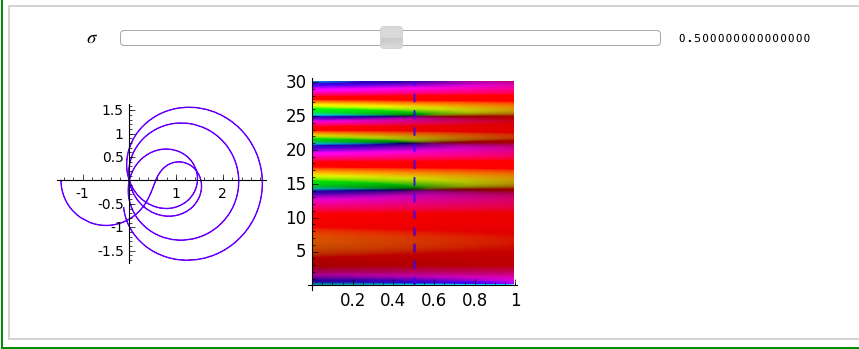# Errors when plotting zeta function parametrically

I have the following piece of code:

def f(x):
return(real_part(zeta(1+x*I)).n())
def g(x):
return(imag_part(zeta(1+x*I)).n())
parametric_plot([f(x),g(x)], (x,2,10))


It should be moderately clear what I'm trying to do - I want to produce a plot of Riemann zeta function on the line Re(z)=1 using parametric plotting. However, when I try to plot this, I get an error TypeError: cannot evaluate symbolic expression numerically. I also tried the same thing without the .n(), but then I get an error TypeError: unable to coerce to a real number. I couldn't find any help online.

It's worth noting that trying to plot function f(x) I get the same error with .n(), but it works just fine without it (as opposed to parametric plot). Does anyone have an idea how to fix the issue?

edit retag close merge delete

Sort by » oldest newest most voted

You mean something like this?In doing a lot of things like this in this project I had to use things lambda t: zeta(sig+t*i).real() because the symbolic version wouldn't handle it, I guess. So lambda functions and .real().

more

1

Thank you very much! I am relatively new to both Python and Sage, so any help is appreciated. By the way (although this isn't strictly relate to my overall question), do you know of a way to make the the plot non-monochromatic? I am thinking of making the hue of the line changing as x increases, so that the plot looks rainbow-y.

Hmm, it's certainly possible (see here)but I don't think we've "wrapped" that matplotlib functionality. See https://trac.sagemath.org/ticket/21564try like this:

f=lambda x: zeta(1+x*I).real_part()
g=lambda x: zeta(1+x*I).imag_part()
parametric_plot([f,g], (x,2,10))

more

Thank you very much! I am relatively new to both Python and Sage, so any help is appreciated.